## 7.7.2 Multiple-Robot Optimality

Suppose that there are two robots as shown in Figure 7.42. There is just enough room to enable the robots to translate along the corridors. Each would like to arrive at the bottom, as indicated by arrows; however, only one can pass at a time through the horizontal corridor. Suppose that at any instant each robot can either be on or off. When it is on, it moves at its maximum speed, and when it is off, it is stopped.7.4Now suppose that each robot would like to reach its goal as quickly as possible. This means each would like to minimize the total amount of time that it is off. In this example, there appears to be only two sensible choices: 1)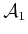stays on and moves straight to its goal while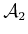is off just long enough to letpass, and then moves to its goal. 2) The opposite situation occurs, in whichstays on andmust wait. Note that when a robot waits, there are multiple locations at which it can wait and still yield the same time to reach the goal. The only important information is how long the robot was off.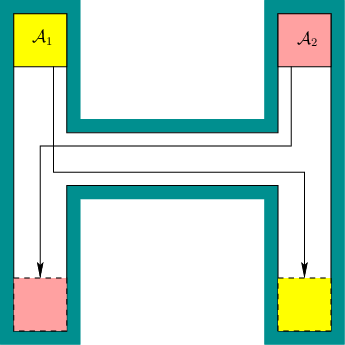Thus, the two interesting plans are that eitheris off for some amount of time,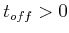, oris off for time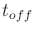. Consider a vector of costs of the form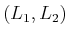, in which each component represents the cost for each robot. The costs of the plans could be measured in terms of time wasted by waiting. This yields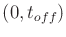and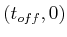for the cost vectors associated with the two plans (we could equivalently define cost to be the total time traveled by each robot; the time on is the same for both robots and can be subtracted from each for this simple example). The two plans are better than or equivalent to any others. Plans with this property are called Pareto optimal (or nondominated). For example, ifwaitssecond too long forto pass, then the resulting costs are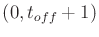, which is clearly worse than. The resulting plan is not Pareto optimal. More details on Pareto optimality appear in Section 9.1.1.

Another way to solve the problem is to scalarize the costs by mapping them to a single value. For example, we could find plans that optimize the average wasted time. In this case, one of the two best plans would be obtained, yieldingaverage wasted time. However, no information is retained about which robot had to make the sacrifice. Scalarizing the costs usually imposes some kind of artificial preference or prioritization among the robots. Ultimately, only one plan can be chosen, which might make it seem inappropriate to maintain multiple solutions. However, finding and presenting the alternative Pareto-optimal solutions could provide valuable information if, for example, these robots are involved in a complicated application that involves many other time-dependent processes. Presenting the Pareto-optimal solutions is equivalent to discarding all of the worse plans and showing the best alternatives. In some applications, priorities between robots may change, and if a scheduler of robots has access to the Pareto-optimal solutions, it is easy to change priorities by switching between Pareto-optimal plans without having to generate new plans each time.

Now the Pareto-optimality concept will be made more precise and general. Suppose there are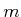robots,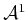,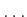,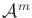. Let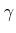refer to a motion plan that gives the paths and timing functions for all robots. For each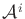, let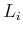denote its cost functional, which yields a value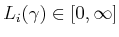for a given plan,. An-dimensional vector,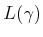, is defined as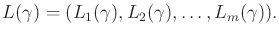(7.28)

Two plans,and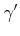, are called equivalent if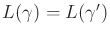. A planis said to dominate a planif they are not equivalent and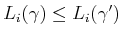for allsuch that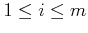. A plan is called Pareto optimal if it is not dominated by any others. Since many Pareto-optimal plans may be equivalent, the task is to determine one representative from each equivalence class. This will be called finding the unique Pareto-optimal plans. For the example in Figure 7.42, there are two unique Pareto-optimal plans, which were already given.

Subsections
Steven M LaValle 2020-08-14